Domain

The domain of a function is the set of values of the independent variable (x) for which the function is defined.

There are two common mathematical operations that are undefined: square root of a negative number and division by zero.

Because a square root cannot successfully act on a negative number, the domain of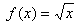is all real numbers x such that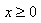. In set notation, this would be written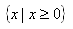.

Division by zero is undefined; therefore values for x that produce a zero denominator are excluded from the domain. For instance, the function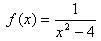has a domain of all real numbers except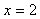and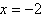. In set notation, this would be written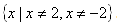.